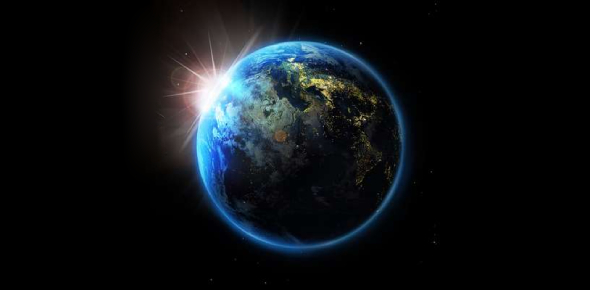Earth Science A 9th Grade Post- Quiz

10 Questions | Total Attempts: 414SettingsThis is the Earth Science A 9th grade post quiz. This is the same quiz your took for your pre-test in this course. The points will count. There is 10 possible points. This is NOT connected to the LMS grade book, so you will have to wait till I manually transfer your grade. You will still need to take: Earth Science A semester exam.

• 1.
A chart showing the amount of rain each month in a region is an example of a scientist's_________________. (AZ HS Science S1:C2:PO5 and 9-10.RH7)
• A.

Variables

• B.

Inferences

• C.

Data

• D.

Conclusions

• 2.
A scientific explanation that can be tested by observation or experiment is a(n)__________. (AZ HS Science S1:C1:PO3)
• A.

Inference

• B.

Simulation

• C.

Variable

• D.

Hypothesis

• 3.
A chart showing the amount of rain each month in a region is an example of a scientist's_________________. (AZ HS Science S1:C2:PO5 and 9-10.RH7)
• A.

Variables

• B.

Inferences

• C.

Data

• D.

Conclusions

• 4.
A scientific explanation that can be tested by observation or experiment is a(n)__________. (AZ HS Science S1:C1:PO3)
• A.

Inference

• B.

Simulation

• C.

Variable

• D.

Hypothesis

• 5.
What type of scientist studies the solid Earth? (AZ HS Science S2:C2:PO1)
• A.

Geologist

• B.

Oceanographer

• C.

Astronomer

• D.

Meteorolgist

• 6.
What is the hardest known mineral? (AZ HS Science S6:C1:PO1)
• A.

Talc

• B.

Quartz

• C.

Diamond

• D.

Gold

• 7.
The technique in which scientists calculate the age of a sample based on the amount of meaning radioactive isotopes it contains is called _______________.  (AZ HS Science S6:C2:PO8)
• A.

Fossil record

• B.

Relative dating

• C.

Miller-Urey experiment

• D.

• 8.
If the Coast Guard warns of a giant wave of water approaching the shore as a result of a major earthquake, they are warning of ___________________. (AZ HS Science S6:C1:PO4)
• A.

An after shock

• B.

Liquefaction

• C.

A tsunami

• D.

Landslides

• 9.
A large stream of moving water that flows through the oceans is called a(n) _____________. (AZ HS Science S6:C2:PO1)
• A.

Current

• B.

Wave

• C.

Tide

• D.

Undertow

• 10.
The diagram above shows four layers of Earth’s atmosphere. Which of the following correctly labels the layers represented by A, B, C, and D (from Earth moving upward) in the correct sequence? (AZ HS Science S6:C2:PO15)
• A.

Troposphere, stratosphere, mesosphere, thermosphere

• B.

Thermosphere, mesosphere, stratosphere, troposphere

• C.

Troposphere, mesosphere, thermosphere, stratosphere

• D.

Mesosphere, troposphere, thermosphere, stratosphere

Related TopicsBack to top